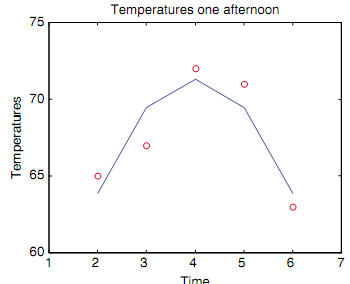## Calling of function polyval, MATLAB in Engineering

Assignment Help:

Calling of Function polyval:

The curve does not appear very smooth on this plot, but that is as there are only five points in the x vector.To estimate the temperature at various times, polyval can be used for discrete x points; it does not have to be used with the whole x vector. For illustration, to interpolate between the given data points and estmate what the temperature was at 2:30 p.m., 2.5 would be used.

>> polyval(coefs,2.5)

ans =

67.1357

The polyval can also be used to extrapolate beyond the given data points, for illustration, to estimate the temperature at 1 p.m.:

>> polyval(coefs,1)

ans =

54.6000

#### Showing cell array elements and attributes, Referring to and Showing Cell A...

Referring to and Showing Cell Array Elements and Attributes: Just as with the other vectors, we can refer to individual elements of the cell arrays. The only difference is tha

#question.

#### Illustration of symbolic variable, Illustration of symbolic variable: ...

Illustration of symbolic variable: When, on the other hand, z is a symbolic variable to start with, quotes are not required around the expression, and the words are automatica

#### Algorithm for subfunction, Algorithm for subfunction: The algorithm fo...

Algorithm for subfunction: The algorithm for subfunction askforn is as shown:  Prompt the user for the positive integer n.  Loop to print an error message and reprom

#### Inverse of square matrix, Inverse of square matrix: The inverse is, he...

Inverse of square matrix: The inverse is, hence the result of multiplying the scalar 1/D by each and every element in the preceding matrix. Note that this is not the matrix A,

#### Algorithm for the function explaine, Algorithm for the function explaine: ...

Algorithm for the function explaine: The algorithm for the function explaine is as shown:  Print a description of e, the exp function, and how to find the approximate va

#### Image processing, Image Processing: The Images are represented as grid...

Image Processing: The Images are represented as grids, or matrices, of picture elements (known as pixels). In MATLAB an image usually is represented as a matrix in which each

#### Illustration of preallocating a vector, Illustration of Preallocating a Vec...

Illustration of Preallocating a Vector: Illustration of calling the function: >> myveccumsum([5 9 4]) ans =     5  14  18 At the first time in the loop, outvec wil

#### Example of exponential function modular program, Example of Exponential fun...

Example of Exponential function modular program: In order to view the distinction in the approximate value for e as n increases, the user kept choosing Limit & entering larger

#### Tracing of square matrices, Tracing of Square matrices: The trace of a...

Tracing of Square matrices: The trace of a square matrix is the addition of all the elements on the diagonal. For illustration, for the preceding matrix it is 1 + 6 + 11 + 16,